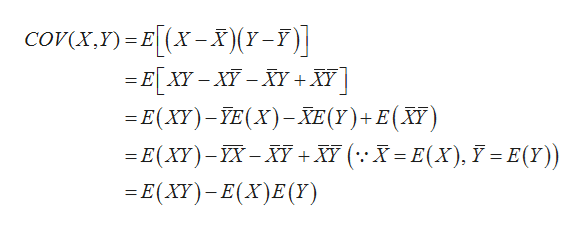# Show that: (a) Cov(X,Y) = E[XY] – E[X]E[Y].(b) If X and Y are independent, then fY|X(y|x) = fY(y)

Question
13 views

Show that: (a) Cov(X,Y) = E[XY] – E[X]E[Y].

(b) If X and Y are independent, then fY|X(y|x) = fY(y)

check_circle

Step 1

Solution:

a.Show that COV(X,Y)=E(XY)–E(X)E(Y)

...help_outlineImage TranscriptionclosecoY(X, Y) = E[x-χj(7-7)] (Χ - E[Y-- Υ+ N] - Ε(W) - (X) - ΣΕ(Υ) + Ε(Y) E(X) - X-N +Y (:X=Ε(Χ), F=E(Υ)) E(XY) -Ε(Χ)Ε (Υ) fullscreen

### Want to see the full answer?

See Solution

#### Want to see this answer and more?

Solutions are written by subject experts who are available 24/7. Questions are typically answered within 1 hour.*

See Solution
*Response times may vary by subject and question.
Tagged in

### Random Variables